SLVS576B SEPTEMBER   2005  – January 2016

PRODUCTION DATA.

1. Features
2. Applications
3. Description
4. Revision History
5. Pin Configuration and Functions
6. Specifications
7. Detailed Description
1. 7.1 Overview
2. 7.2 Functional Block Diagram
3. 7.3 Feature Description
1. 7.3.1 Boost Converter
2. 7.3.2 Negative Charge Pump
3. 7.3.3 Positive Charge Pump
4. 7.3.4 Undervoltage Lockout
5. 7.3.5 Power-On Sequencing, DLY1, DLY2
6. 7.3.6 Gate Voltage Shaping
7. 7.3.7 VCOM Buffer
8. 7.3.8 Protection
4. 7.4 Device Functional Modes
8. Application and Implementation
1. 8.1 Application Information
2. 8.2 Typical Application
1. 8.2.1 Design Requirements
2. 8.2.2 Detailed Design Procedure
3. 8.2.3 Application Curves
3. 8.3 System Examples
9. Power Supply Recommendations
10. 10Layout
11. 11Device and Documentation Support
12. 12Mechanical, Packaging, and Orderable Information

#### Package Options

Refer to the PDF data sheet for device specific package drawings

• RGE|24
• PWP|24

## 8 Application and Implementation

NOTE

Information in the following applications sections is not part of the TI component specification, and TI does not warrant its accuracy or completeness. TI’s customers are responsible for determining suitability of components for their purposes. Customers should validate and test their design implementation to confirm system functionality.

### 8.1 Application Information

The TPS65150 device has been designed to provide the input supply voltages for the source drivers and gate drivers plus the voltage for the common plane in LCD display applications. In addition, the device provides a gate voltage shaping function that can be used to modulate the gate drivers' positive supply to reduce image sticking.

### 8.2 Typical Application

Figure 17 shows a typical application circuit for a monitor display powered from a 5-V supply. It generates up to 450  mA at 13.5 V to power the source drivers, and 20 mA at 23 V and –5 V to power the gate drivers.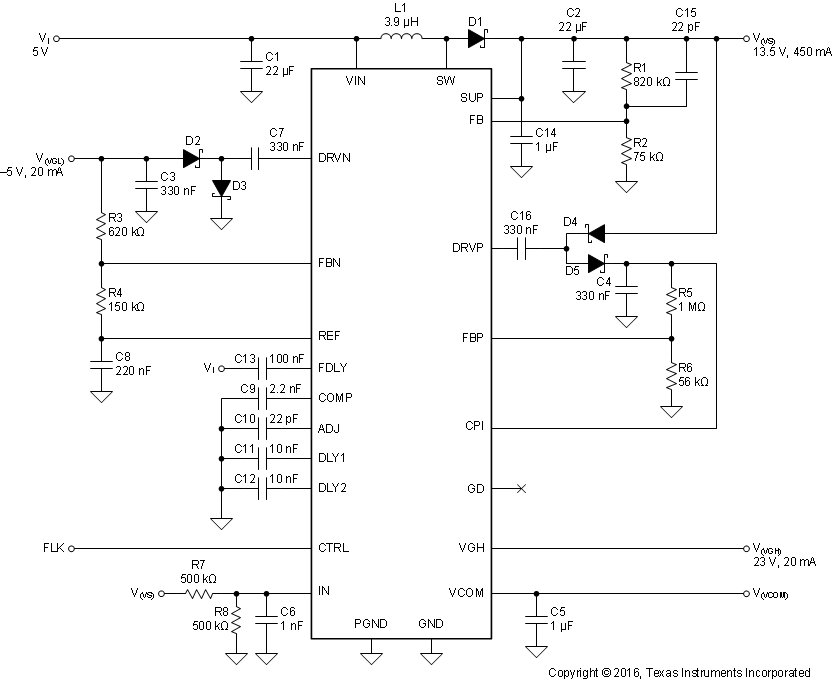Figure 17. Monitor LCD Supply Powered from a 5-V Rail

#### 8.2.1 Design Requirements

Table 2 shows the design parameters for this example.

### Table 2. Design Requirements

PARAMETER SYMBOL VALUE
Input supply voltage VI 5 V
Boost converter output voltage and current V(VS) 13.5 V at 450 mA
Boost converter peak-to-peak output voltage ripple V(VS)(PP) 10 mV
Positive charge pump output voltage and current V(CPI) 23 V at 20 mA
Positive charge pump peak-to-peak output voltage ripple V(VGH)(PP) 100 mV
Negative charge pump output voltage and current V(VGL) –5 V at 20 mA
Negative charge pump peak-to-peak output voltage ripple V(VGL)(PP) 100 mV
Negative charge pump start-up delay time td1 1 ms
Positive charge pump start-up delay time td2 1 ms
Fault delay time td(fault) 45 ms
Gate voltage shaping slope 10 V/µs

#### 8.2.2.1.1 Inductor Selection

Several inductors work with the TPS65150, and with external compensation the performance can be adjusted to the specific application requirements.

The main parameter for the inductor selection is the inductor saturation current, which should be higher than the peak switch current as calculated in Equation 2 with additional margin to cover for heavy load transients. The alternative, more conservative approach, is to choose the inductor with a saturation current at least as high as the maximum switch current limit of 3.4 A.

The second important parameter is the inductor DC resistance. Usually, the lower the DC resistance the higher the efficiency. It is important to note that the inductor DC resistance is not the only parameter determining the efficiency. For a boost converter, where the inductor is the energy storage element, the type and material of the inductor influences the efficiency as well. Especially at a switching frequency of 1.2 MHz, inductor core losses, proximity effects, and skin effects become more important. Usually, an inductor with a larger form factor gives higher efficiency. The efficiency difference between different inductors can vary from 2% to 10%. For the TPS65150, inductor values from 3.3 µH and 6.8 µH are a good choice, but other values can be used as well. Possible inductors are shown in Table 3. Equivalent parts can also be used.

### Table 3. Inductor Selection

INDUCTANCE ISAT DCR MANUFACTURER PART NUMBER DIMENSIONS
4.7 µH 2.6 A 54 mΩ Coilcraft DO1813P-472HC 8.89 mm × 6.1 mm × 5 mm
4.2 µH 2.2 A 23 mΩ Sumida CDRH5D28 4R2 5.7 mm × 5.7 mm × 3 mm
4.7 µH 1.6 A 48 mΩ Sumida CDC5D23 4R7 6 mm × 6 mm × 2.5 mm
4.2 µH 1.8 A 60 mΩ Sumida CDRH6D12 4R2 6.5 mm × 6.5 mm × 1.5 mm
3.9 µH 2.6A 20 mΩ Sumida CDRH6D28 3R9 7 mm × 7 mm × 3 mm
3.3 µH 1.9 A 50 mΩ Sumida CDRH6D12 4R2 6.5 mm × 6.5 mm × 1.5 mm

The first step in the design procedure is to verify whether the maximum possible output current of the boost converter supports the specific application requirements. A simple approach is to estimate the converter efficiency, by taking the efficiency numbers from the provided efficiency curves, or use a worst case assumption for the expected efficiency, for example, 75%.

From Figure 19, it can be seen that the boost converter efficiency is about 85% when operating under the target application conditions. Inserting these values into Equation 1 yields

WHITESPACE

Equation 15.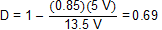WHITESPACE

and from Equation 2, the peak switch current can be calculated as

WHITESPACE

Equation 16.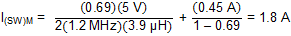WHITESPACE

The peak switch current is the peak current that the integrated switch, inductor, and rectifier diode must be able to handle. The calculation must be done for the minimum input voltage where the peak switch current is highest. For the calculation of the maximum current delivered by the boost converter, it must be considered that the positive and negative charge pumps as well as the VCOM buffer run from the output of the boost converter as well.

#### 8.2.2.2 Rectifier Diode Selection

The rectifier diode reverse voltage rating should be higher than the maximum output voltage of the converter (13.5 V in this application); its average forward current rating should be higher than the maximum boost converter output current of 450 mA, and its repetitive peak forward current should be greater than or equal to the peak switch current of 1.8 A. Not all diode manufacturers specify repetitive peak forward current; however, a diode with an average forward current rating of 1 A or higher is suitable for most practical applications.

From Equation 5, the power dissipated in the rectifier diode is given by

WHITESPACE

Equation 17.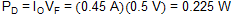WHITESPACE

Table 4 lists a number of suitable rectifier diodes, any of which would be suitable for this application. Equivalent parts can also be used.

### Table 4. Rectifier Diode Selection

IF(AV) VR VF MANUFACTURER PART NUMBER
2 A 20 V 0.44 V at 2 A Vishay Semiconductor SL22
2 A 20 V 0.5 V at 2 A Fairchild Semiconductor SS22
1 A 30 V 0.44 V at 2 A Fairchild Semiconductor MBRS130L
1 A 20 V 0.45 V at 1 A Microsemi UPS120
1 A 20 V 0.45 V at 1 A ON Semiconductor MBRM120

#### 8.2.2.3 Setting the Output Voltage

Rearranging Equation 3 and inserting the application parameters, we get

WHITESPACE

Equation 18.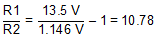WHITESPACE

Standard values of R1 = 820 kΩ and R2 = 75 kΩ result in a nominal output voltage of 13.68 V and satisfy the recommendation that the value R1 be lower than 1 MΩ.

#### 8.2.2.4 Output Capacitor Selection

For best output voltage filtering, a low ESR output capacitor is recommended. Ceramic capacitors have a low ESR value, but tantalum capacitors can be used as well, depending on the application. A 22-µF ceramic output capacitor works for most applications. Higher capacitor values can be used to improve the load transient regulation. See Table 5 for the selection of the output capacitor.

Rearranging Equation 6 and inserting the application parameters, the minimum value of output capacitance is given by Equation 19.

WHITESPACE

Equation 19.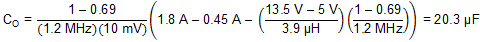WHITESPACE

The closest standard value is 22 µF. In practice, TI recommends connecting an additional 1-µF capacitor directly to the SUP pin to ensure a clean supply to the internal circuitry that runs from this supply voltage.

#### 8.2.2.5 Input Capacitor Selection

For good input voltage filtering, low ESR ceramic capacitors are recommended. A 22-µF ceramic input capacitor is sufficient for most applications. For better input voltage filtering, this value can be increased. See Table 5 for input capacitor recommendations. Equivalent parts can also be used.

### Table 5. Input and Output Capacitance Selection

CAPACITANCE VOLTAGE RATING MANUFACTURER PART NUMBER SIZE
22 µF 16 V Taiyo Yuden EMK325BY226MM 1206
22 µF 6.3 V Taiyo Yuden JMK316BJ226 1206

#### 8.2.2.6 Compensation

From Table 1, it can be seen that the recommended values for C9 and R9 when VI = 5 V are 2.2 nF and 0 Ω respectively, and that a feedforward zero at 11.2 kHz should be added.

Rearranging Equation 7, we get

WHITESPACE

Equation 20.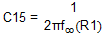WHITESPACE

Inserting fco = 11.2 kHz and R1 = 820 kΩ, we get

WHITESPACE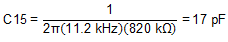WHITESPACE

In this case, a standard value of 22 pF was used.

#### 8.2.2.7.1 Choosing the Output Capacitance

Rearranging Equation 9 and inserting the application parameters, the minimum recommended value of C3 is given by

WHITESPACE

Equation 21.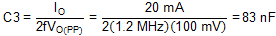WHITESPACE

In this application, a capacitance of 330 nF was used to allow the same value to be used for all charge pump capacitances.

#### 8.2.2.7.2 Choosing the Flying Capacitance

A minimum flying capacitance of 100 nF is recommended. In this application, a capacitance of 330 nF was used to allow the same value to be used for all charge pump capacitances.

#### 8.2.2.7.3 Choosing the Feedback Resistors

From Equation 22, the ratio of R3 to R4 required to generate an output voltage of –5 V is given by

WHITESPACE

Equation 22.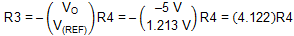WHITESPACE

Values of R3 = 620 kΩ and R4 = 150 kΩ generate a nominal output voltage of –5.014 V and load the REF pin with only 8 µA.

#### 8.2.2.7.4 Choosing the Diodes

The average forward current in D2 and D3 is equal to the output current and therefore a maximum of 20 mA. The peak repetitive forward current in D2 and D3 is equal to twice the output current and therefore less than 40 mA..

The BAT54S comprises two Schottky diodes in a small SOT-23 package and easily meets the current requirements of this application.

#### 8.2.2.8.1 Choosing the Flying Capacitance

A minimum flying capacitance of 330 nF is recommended.

#### 8.2.2.8.2 Choosing the Output Capacitance

Rearranging Equation 10 and inserting the application parameters, we get

WHITESPACE

Equation 23.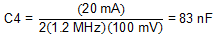WHITESPACE

In this application, a nominal value of 330 nF was used to allow the same value to be used for all charge pump capacitances.

#### 8.2.2.8.3 Choosing the Feedback Resistors

Rearranging Equation 8 and inserting the application parameters, we get

WHITESPACE

Equation 24.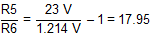WHITESPACE

Standard values of 1 MΩ and 56 kΩ result in a nominal output voltage of 22.89 V.

#### 8.2.2.8.4 Choosing the Diodes

The average forward current in D4 and D5 is equal to the output current and therefore a maximum of 20 mA. The peak repetitive forward current in D4 and D5 is equal to twice the output current and therefore less than 40 mA.

#### 8.2.2.9 Gate Voltage Shaping

Rearranging Equation 13 and inserting I(ADJ) = 200 µA and slope = 10 V/µs, we get

WHITESPACE

Equation 25.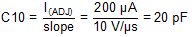WHITESPACE

The closest standard value for C10 is 22 pF.

#### 8.2.2.10 Power-On Sequencing

Rearranging Equation 12 and inserting td1 = td2 = 1 ms and Vref2 = 1.213 V, we get

WHITESPACE

Equation 26.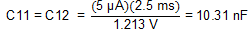WHITESPACE

10 nF is the closest standard value.

#### 8.2.2.11 Fault Delay

Rearranging Equation 14 and inserting td(FDLY) = 45 ms, we get

WHITESPACE

Equation 27.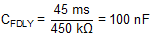WHITESPACE

100 nF is a standard value.

#### 8.2.2.12 Undervoltage Lockout Function

The TPS65150 device contains an undervoltage lockout (UVLO) function that stops the device operating if the voltage on the VDD pin is too low.

#### 8.2.3 Application Curves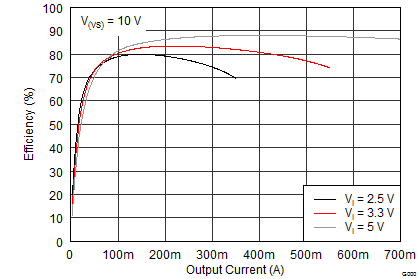I(VGH) = 0 mA I(VGL) = 0 mA
Figure 18. Boost Converter Efficiency (V(VS) = 10 V)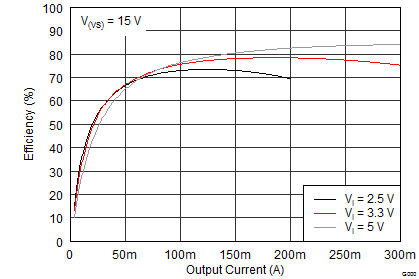I(VGH) = 0 mA I(VGL) = 0 mA
Figure 20. Boost Converter Efficiency (V(VS) = 15 V)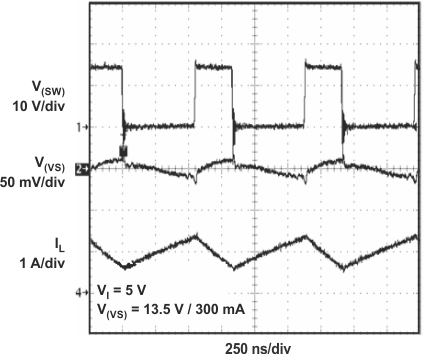Figure 22. Boost Converter Operation (Nominal Load)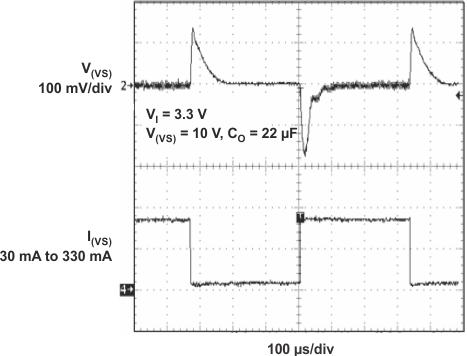Figure 24. Boost Converter Load Transient Response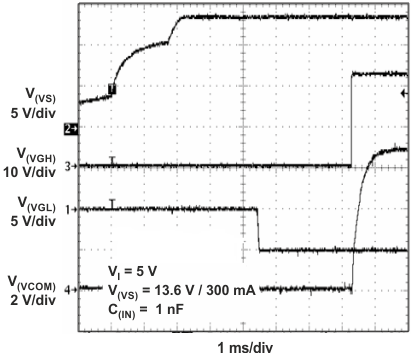Figure 26. Power-On Sequencing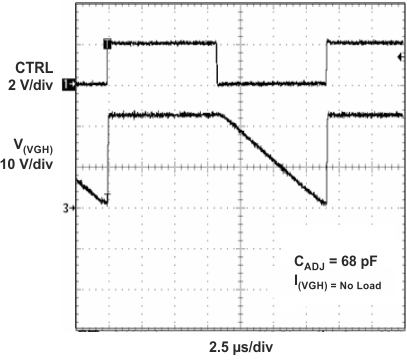Figure 28. Gate Voltage Shaping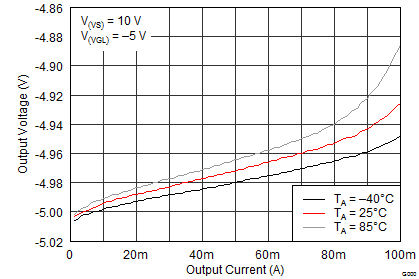Figure 30. Negative Charge Pump Load Regulation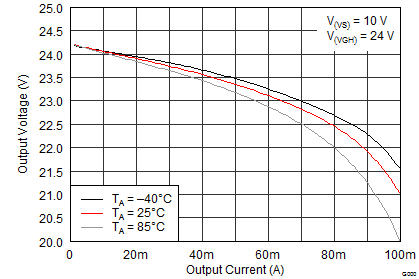Figure 32. Positive Charge Pump Load Regulation (×3)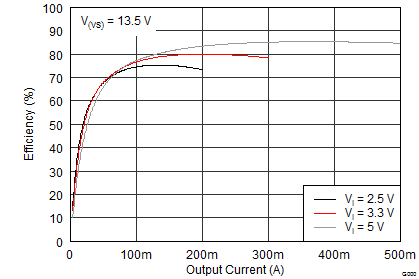I(VGH) = 0 mA I(VGL) = 0 mA
Figure 19. Boost Converter Efficiency (V(VS) = 13.5 V)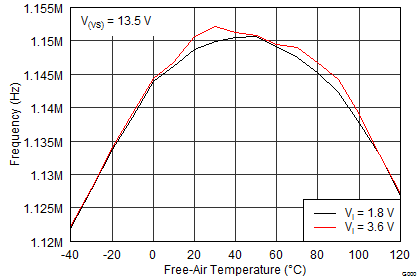Figure 21. Boost Converter Switching Frequency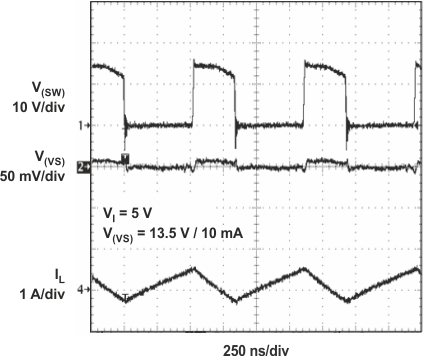Figure 23. Boost Converter Operation (Light Load)Figure 25. Boost Converter Soft Start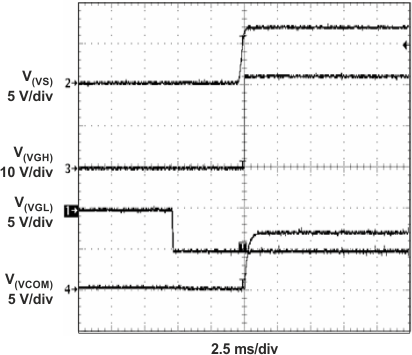Figure 27. Power-On Sequencing With External Isolation MOSFETFigure 29. Adjustable Fault Detection Time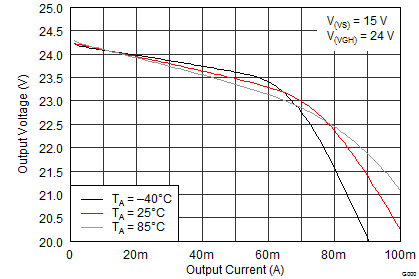Figure 31. Positive Charge Pump Load Regulation (×2)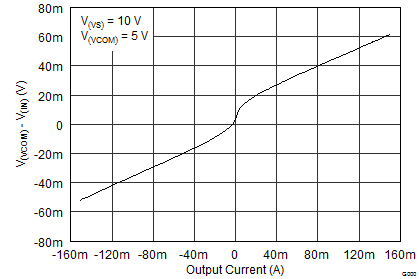Figure 33. VCOM Buffer Load Regulation

### 8.3 System ExamplesFigure 34. Notebook LCD Supply Powered from a 2.5-V RailFigure 35. Monitor LCD Supply Powered from a 5-V Rail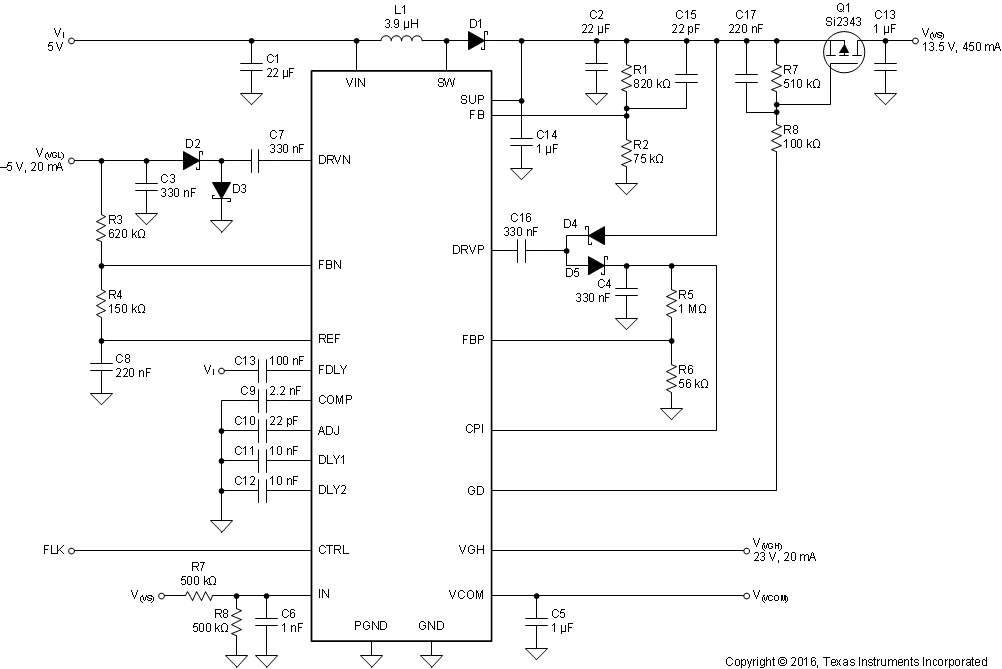Figure 36. Typical Isolation and Short Circuit Protection Switch for V(VS) Using Q1 and Gate Drive Signal (GD)